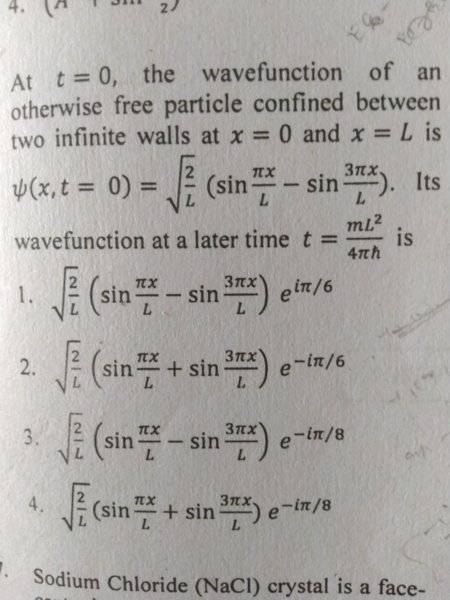# Time evolution of wave function in an infinite square well potential

• Apashanka
ApashankaFor this problem at t=0
Ψ(x,0)=Ψ13
Where Ψ1 and Ψ3are the normalised eigenstates corresponding to energy level 1 and 3 of the infinite square well potential.
Now for it's time evolution it will be Ψ1exp(-iE1t/ħ)- Ψ3exp(-iE3t/ħ)
And taking the time given in the question the time part of the individual states is coming to be exp(-iπ/8) and exp(-9iπ/8) respectively which is equivalent to (-1)^(-1/8) and (-1)^(-9/8) respectively and the two are equal
Hence in my opinion the ans is option 3
But the correct option is given option 4??
Am I right?

#### Attachments

Homework Helper
Gold Member
2022 Award
View attachment 235705
For this problem at t=0
Ψ(x,0)=Ψ13
Where Ψ1 and Ψ3are the normalised eigenstates corresponding to energy level 1 and 3 of the infinite square well potential.
Now for it's time evolution it will be Ψ1exp(-iE1t/ħ)- Ψ3exp(-iE3t/ħ)
And taking the time given in the question the time part of the individual states is coming to be exp(-iπ/8) and exp(-9iπ/8) respectively which is equivalent to (-1)^(-1/8) and (-1)^(-9/8) respectively and the two are equal
Hence in my opinion the ans is option 3
But the correct option is given option 4??
Am I right?

You look right to me. Note also that the wavefunctions given are not normalised.

Homework Helper
Gold Member
(-1)^(-1/8) and (-1)^(-9/8) respectively and the two are equal
These are not quite equal.

•PeroK
Apashanka
These are not quite equal.
Thanks

Homework Helper
Gold Member
2022 Award
Thanks

The problem with ##\pi## is that ##\pi## radians ought to be a full circle. But, it's not. Instead ##2\pi## radians is a full circle. You always have to be careful with this. I make this mistake all the time: especially in the case that ##\pi/4## is not a right angle. I always think ##\pi/4## should be a right angle, but it's not.

There's actually a semi-serious movement in maths education to replace ##\pi## with ##\tau## where ##\tau = 2\pi##. Then ##\tau## would be a full circle and ##\tau/4## would be a right angle. I'd move to ##\tau## tomorrow if I could.

Sorry for misleading you. My mistake.

Apashanka
The problem with ##\pi## is that ##\pi## radians ought to be a full circle. But, it's not. Instead ##2\pi## radians is a full circle. You always have to be careful with this. I make this mistake all the time: especially in the case that ##\pi/4## is not a right angle. I always think ##\pi/4## should be a right angle, but it's not.

There's actually a semi-serious movement in maths education to replace ##\pi## with ##\tau## where ##\tau = 2\pi##. Then ##\tau## would be a full circle and ##\tau/4## would be a right angle. I'd move to ##\tau## tomorrow if I could.

Sorry for misleading you. My mistake.
I have used the fact that exp(imπ)={exp(iπ)}^m={cos(π)+i*sin(π)}^m=(-1)^m
Where m can be both positive and negative real number.
Will you please explain whether it is justifiable ,I want to clarify??
Thanks
I have used this fact by which exp(-iπ/8) =(-1)^(-1/8) and exp (-9iπ/8)=(-1)^(-9/8) and the two are equal.

Last edited:
Homework Helper
Gold Member
2022 Award
I have used the fact that exp(imπ)={exp(iπ)}^m={cos(π)+i*sin(π)}^m=(-1)^m
Where m can be both positive and negative real number.
Will you please explain whether it is justifiable ,I want to clarify??
Thanks
I have used this fact by which exp(-iπ/8) =(-1)^(-1/8) and exp (-9iπ/8)=(-1)^(-9/8) and the two are equal.

##\exp(\frac{-i\pi}{8}) = \exp(\frac{-i17\pi}{8}) \ne \exp(\frac{-i9\pi}{8})##

The period of ##\exp, \sin, \cos## is ##2 \pi##, not ##\pi##.

Homework Helper
Gold Member
I have used this fact by which exp(-iπ/8) =(-1)^(-1/8) and exp (-9iπ/8)=(-1)^(-9/8) and the two are equal.
What do you get if you divide exp (-iπ/8) by exp(-9iπ/8)? First reduce exp (-iπ/8) ##\div## exp(-9iπ/8) to a single exponential. Likewise, what does (-1)^(-1/8) ##\div## (-1)^(-9/8) reduce to?

Apashanka
##\exp(\frac{-i\pi}{8}) = \exp(\frac{-i17\pi}{8}) \ne \exp(\frac{-i9\pi}{8})##

The period of ##\exp, \sin, \cos## is ##2 \pi##, not ##\pi##.
Ohh thanks sir for the clarification .
But sir will you please explain how I am getting the two to be same which I have posted above that remains a confusion??

Apashanka
What do you get if you divide exp (-iπ/8) by exp(-9iπ/8)? First reduce exp (-iπ/8) ##\div## exp(-9iπ/8) to a single exponential. Likewise, what does (-1)^(-1/8) ##\div## (-1)^(-9/8) reduce to?
Yes sir that's coming to be -1
Thanks sir